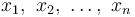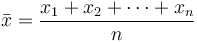# Mean vs Median

## Definitions of mean and median

In mathematics and statistics, the mean or the arithmetic mean of a list of numbers is the sum of the entire list divided by the number of items in the list. When looking at symmetric distributions, the mean is probably the best measure to arrive at central tendency. In probability theory and statistics, a median is that number separating the higher half of a sample, a population, or a probability distribution, from the lower half.

## How to calculate

The Mean or average is probably the most commonly used method of describing central tendency. A mean is computed by adding up all the values and dividing that score by the number of values. The arithmetic mean of a sample$x_1,\ x_2,\ \ldots,\ x_n$ is the sum the sampled values divided by the number of items in the sample:$\bar{x} = \frac{x_1+x_2+\cdots +x_n}{n}$

The Median is the number found at the exact middle of the set of values. A median can be computed by listing all numbers in ascending order and then locating the number in the center of that distribution. This is applicable to an odd number list; in case of an even number of observations, there is no single middle value, so it is a usual practice to take the mean of the two middle values.

### Example

Let us say that there are nine students in a class with the following scores on a test: 2, 4, 5, 7, 8, 10, 12, 13, 83. In this case the average score (or the mean) is the sum of all the scores divided by nine. This works out to 144/9 = 16. Note that even though 16 is the arithmetic average, it is distorted by the unusually high score of 83 compared to other scores. Almost all of the students’ scores are below the average. Therefore, in this case the mean is not a good representative of the central tendency of this sample.

The median, on the other hand, is the value which is such that half the scores are above it and half the scores below. So in this example, the median is 8. There are four scores below and four above the value 8. So 8 represents the mid point or the central tendency of the sample.

Credits:”Mean vs Median.” Diffen.com. Diffen LLC, n.d. Web. 21 Apr 2016. < http://www.diffen.com/difference/Mean_vs_Median >